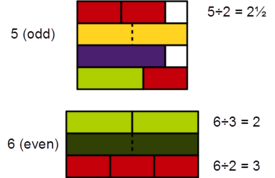# Odd number facts for kids

Kids Encyclopedia FactsCuisenaire rods: 5 (yellow) cannot be evenly divided in 2 (red) by any 2 rods of the same color/length, while 6 (dark green) can be evenly divided in 2 by 3 (light green).

An odd number is an integer which is not a multiple of two. If it is divided by two the result is a fraction. One is the first odd positive number. The next four bigger odd numbers are three, five, seven, and nine. So some sequential odd numbers are: {1,3,5,7,9,11,13,15,17,19,21,23,25...}

An integer that is not an odd number is an even number. Some sequential even numbers are: {0,2,4,6,8,10,12,14,16...}. An even number is defined as a whole number that is a multiple of two. If an even number is divided by two, the result is another whole number. On the other hand, an odd number, when divided by two, will result in a fraction.

Since odd and even numbers are defined only in reference to the set of integers (..., −3, −2, −1, 0, 1, 2, 3, ...), all negative numbers can also be either odd or even.

To find the set of odd natural numbers, we use 2N+1 where N is any integer.Odd number Facts for Kids. Kiddle Encyclopedia.